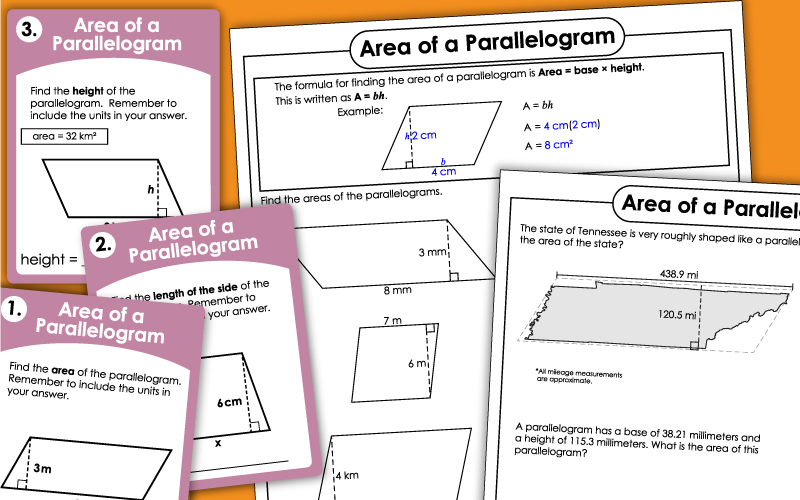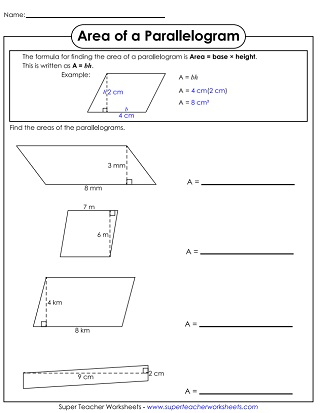# Areas of Parallelograms

This page has a collection of printables that can be used to teach and review areas of parallelograms.## Basic Level:Single-Digit Measurements

Use the formula to calculate the areas of the four parallelograms shown. This basic version contains single-digit, whole number measurements.
This page has nine parallelogram illustrations. Multiply the base times the height to find the area of each.
This worksheet has three parts. In the first part, calculate the base and height of the parallelograms. In the second part, students use subtraction to find the area of the unshaded parts of a shape. In the final part, they calculate the area of an irregular shape.
Download a deck of 30 Area of a Parallelogram cards. Use them for peer study groups, classroom scavenger hunts, or small group instruction.
Find the area and perimeter of each of the nine parallelograms given.

## Level: IntermediateDouble-Digit Measurements

Use the formula to calculate the area of the parallelograms shown. In the intermediate-level worksheets, the measurements are mostly 2-digit whole numbers.
Here's a worksheet with 9 parallelogram pictures. Calculate the area of each by multiplying base times height.
Use higher-order thinking and calculate answers to these four challenging questions.
This math card set has 30 parallelogram graphics that can be used for peer tutoring, learning centers, document camera examples, or exit slips.
On this worksheet, your students will find both the perimeter and area of the shapes.

## Level: AdvancedDecimal & Fraction Measurements

On this advanced level worksheet, students will find the area of 5 different parallelograms using 3- and 4-digit numbers and decimals.
Use the formula A=bh to find the areas of the 9 parallelograms shown.
Use reasoning and higher thinking skills to solve these problems.
These task cards can be used on your document projector, for whole-class games, as exit slips, or for daily practice.
Calculate both the perimeter and area of these parallelograms. Side and height lengths include decimals and fractions.
More Area Worksheets

Find the areas of all types of shapes, including circles, rectangles, and triangles. There's a section for surface area worksheets too.

Geometry Worksheets

Link to printables on symmetry, tessellations, translation/rotation/reflection, polygons, plotting points, and more.

## Worksheet ImagesMy Account
Site Information